# Online Verbal Reasoning Test - Verbal Reasoning Test 2Loading Test...

Instruction:

• This is a FREE online test. DO NOT pay money to anyone to attend this test.
• Total number of questions : 20.
• Time alloted : 30 minutes.
• Each question carry 1 mark, no negative marks.
• DO NOT refresh the page.
• All the best :-).

1.

P started from his house towards west. After walking a distance of 25 m. He turned to the right and walked 10 m. He then again turned to the right and walked 15 m. After this he is to turn right at 135o and to cover 30 m. In which direction should he go?

A.
 WestB.
 SouthC.
 South-WestD.
 South-EastExplanation: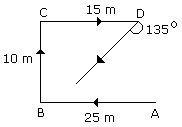Hence he should go in the South-West direction.

2.

One day at 2 P.M. Manisha and Madhuri were talking to each other face to face. If Manisha's shadow was exactly to the left of Madhuri, which direction Manisha was facing?

A.
 NorthB.
 SouthC.
 EastD.
 None of theseExplanation:Manisha's shadow at 2 P.M. is exactly to the left of Madhuri. Then Madhuri should be facing towards South-East.

Hence then Manisha must be facing towards North-east.

At 2 P.M. sun rays are oblique.

Hence shadow will also be oblique.

3.

Veena who is the sister-in-law of Ashok, is the daughter-in-law of Kalyani. Dheeraj is the father of Sudeep who is the only brother of Ashok. How Kalyani is related to Ashok?

A.
 Mother-in-lawB.
 AuntC.
 WifeD.
 None of theseExplanation:

Ashok is the only brother of Sudeep and Veena is the sister-in-law of Ashok. Hence Veena is the wife of Sudeep. Kalyani is the mother-in-law of Veena. Kalyani is the mother of Ashok.

4.

A's son B is married with C whose sister D is married to E the brother of B. How D is related to A?

A.
 SisterB.
 Daughter's-in-lawC.
 Sister-in-lawD.
 CousinExplanation:

Since E is the brother of B

Therefore, A is the father of E

but D is the wife of E.

Hence, D is the daughter-in-law of A.

5.

A boy is sitting at the back seat of a car. When the driver suddenly starts moving the car (in forward direction), the boy experiences a backward force?

A.
 AlwaysB.
 NeverC.
 OftenD.
 SometimesExplanation:

When a car suddenly starts the lower part of the boy's body will be in the motion while his upper will be at rest.

Hence he will always experience backward force:

Direction (for Q.No. 6):
The figure given on the left hand side in each of the following questions is folded to form a box. Choose from the alternatives (1), (2), (3) and (4) the boxes that is similar to the box formed.
6.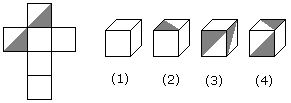A.
 1, 2 and 4 onlyB.
 3 and 4 onlyC.
 1 and 2 onlyD.
 1, 2 and 3 onlyDirection (for Q.No. 7):

Below in each of the questions are given two statements I and II. These statements may be either independent causes or may be effects of independent causes or a common cause. One of these statements may be the effect of the other statements. Read both the statements and decide which of the following answer choice correctly depicts the relationship between these two statements.

• (A) If statement I is the cause and statement II is its effect.
• (B) If statement II is the cause and statement I is its effect.
• (C) If both the statements I and II are independent causes.
• (D) If both the statements I and II are effects of independent causes.
• (E) If both the statements I and II are effects of some common cause.
7.

Statements:

1. Rural and semi-urban areas in the country have been suffering due to load-shedding for quite some time.
2. If the Government is not able to overcome the power crisis, load-shedding will be extended even to the urban areas.

A.
 Statement I is the cause and statement II is its effect.B.
 Statement II is the cause and statement I is its effect.C.
 Both the statements I and II are independent causes.D.
 Both the statements I and II are effects of independent causes.E.
 Both the statements I and II are effects of some common cause.Explanation:

If certain preventive measures are practiced at the rural and semi urban areas the problem will be solved. But if the same is not practiced even at the cities the problem may roll into the city also, so the two effects are of some common cause.

Direction (for Q.No. 8):
Each of these questions given below contains three elements. These elements may or may not have some inter linkage. Each group of elements may fit into one of these diagrams at (A), (B), (C), (D) and/or (E). You have to indicate the group of elements which correctly fits into the diagrams.
8.

Which of the following diagrams indicates the best relation between Hockey, Football and Cricket ?

A.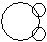B.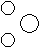C.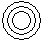D.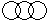Explanation:

All these three games are different from each other.

Direction (for Q.Nos. 9 - 10):
In each of the following questions find out the alternative which will replace the question mark.
9.

Safe : Secure :: Protect : ?

A.
 LockB.
 SureC.
 GuardD.
 ConserveExplanation:

As safe ans secure have same meaning in the same way protect and guard have same meaning.

10.

Ice : Coldness :: Earth : ?

A.
 WeightB.
 JungleC.
 GravitatismD.
 SeaExplanation:

As effect of Ice is coldness similarly the effect of Earth is gravitation.

Direction (for Q.Nos. 11 - 12):
In each of the following questions find out the alternative which will replace the question mark.
11.

Computer : fqprxvht :: Language : ?

A.
 oxpixdigB.
 ocqicyigC.
 ocqixcjgD.
 ocqixcigExplanation: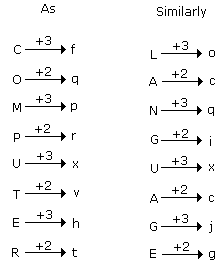12.

? : QEHMDF :: WIDELY : HVCDXK

A.
 FRINGEB.
 STRINGC.
 FRANCED.
 DEMANDExplanation: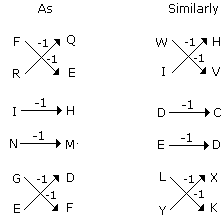Direction (for Q.No. 13):
In each of the following questions find out the alternative which will replace the question mark.
13.

68 : 130 :: ? : 350

A.
 220B.
 224C.
 222D.
 226Explanation:

As, 68 = (4)3 + 4

130 = (5)3 + 5

and 350 = (7)3 + 7

Therefore, ? = (6)3 + 6 = 222

Direction (for Q.No. 14):
In each word of the following questions consists of pair of words bearing a relationship among these, from amongst the alternatives, pick up the pair that best illustrate a similar relationship.
14.

Numismatist : Coins

A.
 Jeweller : JewelsB.
 Cartographer : MapsC.
 Philatelist : StampsD.
 Geneticist : ChromosomesExplanation:

As Numismatist collects coins similarly Philatelist collects stamps.

Direction (for Q.No. 15):
Each of these questions are based on the information given below:
1. A ,B, C, D and E are five men sitting in a line facing to south - while M, N, O, P and Q are five ladies sitting in a second line parallel to the first line and are facing to North.
2. B who is just next to the left of D, is opposite to Q.
3. C and N are diagonally opposite to each other.
4. E is opposite to O who is just next right of M.
5. P who is just to the left of Q, is opposite to D.
6. M is at one end of the line.
15.

If O and P, A and E and B and Q interchange their positions, then who will be the second person to the right of the person who is opposite to the person second of the right of P ?

A.
 DB.
 AC.
 ED.
 OExplanation:

Old arrangement :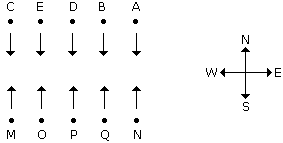New arrangement :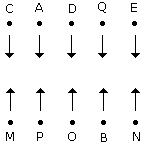Direction (for Q.No. 16):
Six girls are sitting in a circle facing to the centre of the circle. They are P, Q, R, S, T and V. T is not between Q and S but some other one. P is next to the left of V. R is 4th to the right of P.
16.

If P and R interchange their positions then which of the following pair will sit together ?

A.
 RTB.
 PVC.
 VTD.
 QVExplanation:

After changing the position of P and R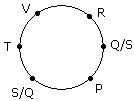V and T are together.

17.

Which one will replace the question mark ?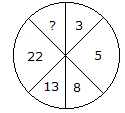A.
 45B.
 29C.
 39D.
 37Explanation: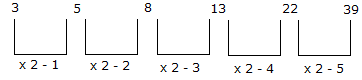18.

Which one will replace the question mark ?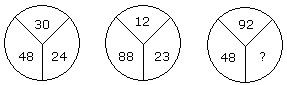A.
 60B.
 46C.
 86D.
 75Explanation:

(30 - 24) x 8 = 48

and (23 - 12) x 8 = 88

Therefore, (92 - 86) x 8 = 48.

19.

Which one will replace the question mark ?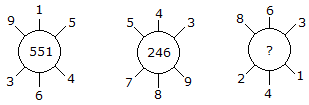A.
 262B.
 622C.
 631D.
 824Explanation:

(915 - 364) = 551

(789 - 543) = 246

(863 - 241) = 622.

Direction (for Q.No. 20):
In each of the following questions, arrange the given words in a meaningful sequence and thus find the correct answer from alternatives.
20.

Arrange the words given below in a meaningful sequence.

 1. Key 2. Door 3. Lock 4. Room 5. Switch on

A.
 5, 1, 2, 4, 3B.
 4, 2, 1, 5, 3C.
 1, 3, 2, 4, 5D.
 1, 2, 3, 5, 4Explanation:

The correct order is :

 Key Lock Door Room Switch on 1 3 2 4 5